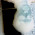### Intuition to convergence

We've all seen these kinds of sums. You start with something obviously divergent, like:

$$S = 1 + 2 + 4 + 8 + ...$$
And then apply standard manipulations on it to obtain a bizarrely finite result:

$$\begin{gathered} \Rightarrow S = 1 + 2(1 + 2 + 4 + ...) \hfill \\ \Rightarrow S = 1 + 2S \hfill \\ \Rightarrow S = - 1 \hfill \\ \end{gathered}$$
How exactly is this result to be interpreted? Surely the definition of an infinite sum is as a limit of a finite sum as the upper limit increases without bound -- by this definition it would seem that $S$ evidently doesn't approach $-1$, it diverges to infinity. Is there, then, something wrong with the form of our argument? And if so, why does it seem to work for so many other sums, like convergent geometric progressions?

We'll get to all that in a moment, but first, let's talk about how to fold a tie into thirds. We know how to fold a tie -- or a strip of paper or a rope or whatever -- into halves, into quarters, into any power of two. But how would one fold it into thirds? Sure, we can approximate it by trial and error, but is there a more efficient algorithm to approximate it?

Here's one way: start with some approximation to 1/3 of the tie -- any approximation, however good or bad. Now consider the rest of the tie (~2/3) and fold it in half. Take one of these halves -- this is demonstrably a better approximation to 1/3 than your original. In fact, the error in this approximation is exactly half the error in the original approximation. You can keep repeating this process, and approach an arbitrarily close value to 1/3.

Why does it work? Well, it's obvious why it works. More interestingly, how could one have come up with this technique from scratch?

The key insight here is that if you had started from exactly 1/3 and performed this algorithm, defined as $x_{n+1}=\frac12(1-x_n)$, the sequence would be constant -- it would be 1/3s all the way down.

However, this is not a sufficient argument. For instance, here's another sequence of which 1/3 is a fixed point: the algorithm $x_{n+1}=1-2x_n$. However here, if you were to start with any other number but 1/3, the sequence would not approach 1/3, but rather diverge away. While 1/3 is still a fixed point, this is an unstable fixed point, while in the previous case it was a stable fixed point.

But what exactly is wrong with extending the same argument to $x_{n+1}=1-2x_n$? Well, perhaps we should state the argument precisely in the case of $x_{n+1}=\frac12(1-x_n)$. The reason we know this converges to 1/3 regardless of the initial value is that 1/3 is the only value which stays the same in the algorithm (i.e. is a steady-state solution). Convergence of the sequence requires that the sequence the fluctuations get smaller, i.e. the sequence approaches a value that doesn't fluctuate around, it approaches a steady state.

But this reveals our central assumption -- we assumed that the sequence is convergent at all! If it is convergent, then 1/3 is the only value it could converge to, because convergence means approaching a steady state, and 1/3 is the only steady state.

The same principle applies to our original problem -- an infinite series is also a sequence, a sequence of partial sums. Our mistake is really in this step:

$$\begin{gathered} ... \hfill \\ 1 + 2(1 + 2 + 4 + 8 + ...) = 1 + 2S \hfill \\ \end{gathered}$$
By declaring that this is the same $S$, we have assumed that this sum really has a value. To be even clearer, consider this (taking $n\to\infty$):

$$\begin{gathered} S = 1 + 2 + 4 + ... + {2^n} \hfill \\ S = 1 + 2(1 + 2 + 4 + ... + {2^{n - 1}}) = 1 + 2S? \hfill \\ \end{gathered}$$
In other words, we assumed that $S$ reaches a steady state, that removing the last term $2^n$ wouldn't change the value of the summation. This would've been true if we were dealing with $(1/2)^n$ instead, because then the partial sum does reach a steady state, since its "derivative", $(1/2)^n$, approaches approaches 0.

With that said, the sum $1+2+4+8+...=-1$ (and other such surprising results) can in fact be correct. What we've proven here is that if the sum converges, it converges to -1. Otherwise, it's $2^\infty -1$. If you can construct an axiomatic system in which the sum does converge, where 0 behaves like $2^\infty$ in some specific sense, then the identity would be true. Such a system does in fact exist, it's called the 2-adic system.

You know, there is a sense in which you can understand the 2-adic system. When you take partial sums of $1+2+4+8+...$, you always get sums that are "1 less than a power of 2". $1+2+4+8+16=2^5-1$, for example -- what's the significance of $2^5$? Well, it's a number which 2 divides into 5 times. What's a number that 2 divides into an infinite number of times? Well, it's zero, and $0-1=-1$. Ths might sound like a ridiculous argument, and indeed it is false in our conventional algebra system, but the foundation of the 2-adic system.

Explain similarly why $1+3+9+27+...=-1/2$ in the 2-adic system.

The understanding of convergence we gained here -- from the tie example -- was pretty fantastic. It applies to all sorts of infinite sequences -- ordinary recurrences, (such in the form of) infinite series, continued fractions, etc. The idea of stable and unstable fixed points is a general one, and a very important one. Recommended watching:

1.1.